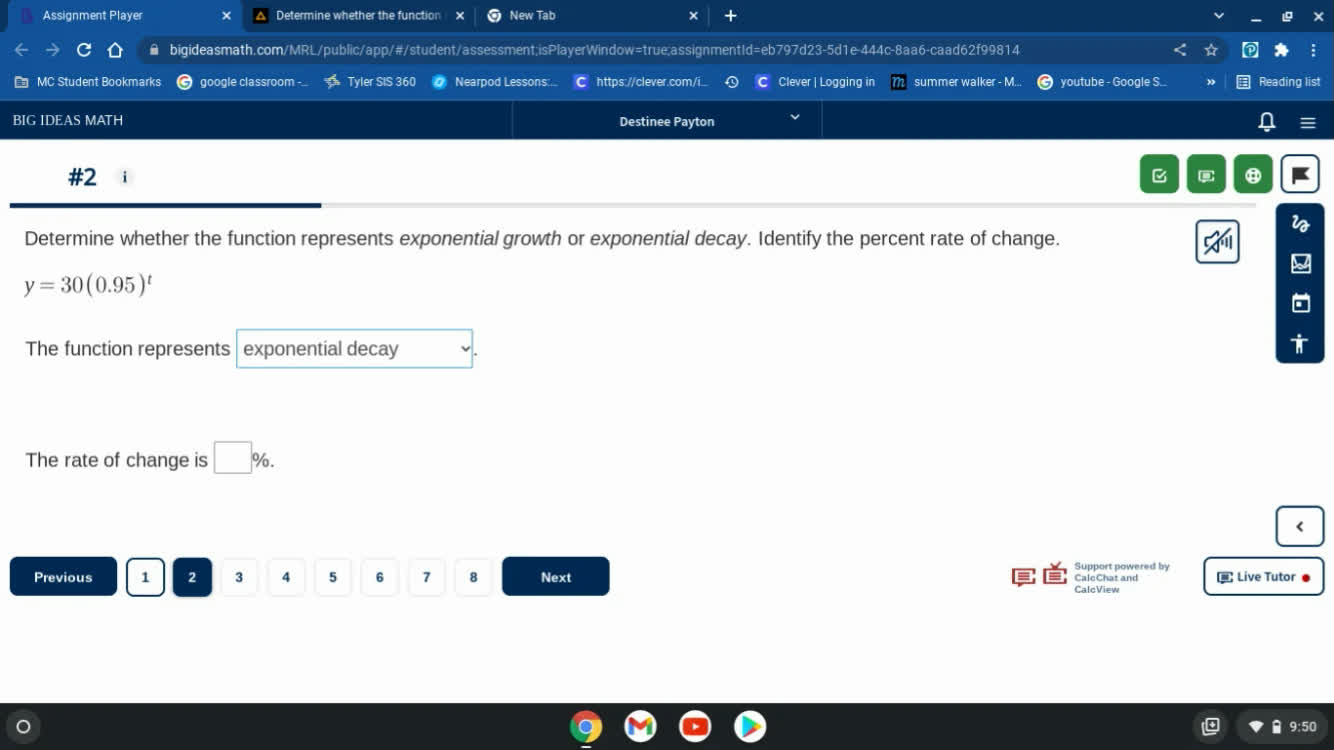# Be Pro in Exponential Growth and Decay Problems

Recent questions in Exponential growth and decayjdkhoo88o8j 2023-02-04

## ______ is the most abundant gas in the atmosphere and makes up 78% of the air.jamstavajo 2022-09-03

## Scientists measure a bacteria population and find that it is 2,700. Three days later, they find that the population has doubled. Write a function f(d) that could describe the bacteria population d days after the scientists first measured it, assuming it grows exponentially?

Algebra IIOpen questionSamuel Herrera Liberato2022-03-30

## There was a sample of300milligrams of a radioactive substance to start a study. Since then, the sample has decayed by2.3%each year. Lettbe the number of years since the start of the study. Letybe the mass of the sample in milligrams. Write an exponential function showing the relationship betweenyandt.MMDCCVIII446 2022-02-14

## Determine whether the function represents exponential growth or exponential decay. Identify the percent rate of change. y=30(0.95)^tgravaviwoa 2022-02-13

## State whether the equation represents exponential growth, exponential decay,f(x)=14⋅12xBenoguigoliB 2021-07-31

## Determine whether the function represents exponential growth or exponential decay. Identify the percent rate of change. Then graph the function. $f\left(x\right)={\left(0.2\right)}^{x}$boitshupoO 2021-07-05

## Determine whether the function represents exponential growth or exponential decay. Identify the percent rate of change. $f\left(t\right)=6\left(0.84{\right)}^{t}$boitshupoO 2021-07-04

## Without graphing, determine whether the function $y=0.3\left(1.25\right)x$ represents exponential growth or decay. State how you made the determination.preprekomW 2021-07-04

## Determine whether the function $y=156{\left(0.825\right)}^{t}$ represents exponential growth, exponential decay, or neither. ExplainAlbarellak 2021-06-27

## Identify each of the following functions as exponential growth or decay. Then give the rate of growth or decay as a percent. $y=a{\left(\frac{1}{2}\right)}^{t}$UkusakazaL 2021-06-23

## Determine whether each function is an example of exponential growth or decay. Then, find the y-intercept. $y=25/7\left(7/5{\right)}^{x}$Cabiolab 2021-06-22

## Find the multiplier for the rate of exponential growth or decay. 0.4% growth _______Jerold 2021-06-21

## Determine whether each function is an example of exponential growth or decay. Then find the y-intercept. $y=\frac{7}{8}{\left(18\right)}^{x}$Marvin Mccormick 2021-06-21

## Identify the function as exponential growth or exponential decay.Then identify the growth or decay factor. $y=1.3{\left(\frac{1}{4}\right)}^{x}$geduiwelh 2021-06-21
## For the following exercises, determine whether the equation represents exponential growth, exponential decay, or neither. Explain. $y=220\left(1.06{\right)}^{x}$Aneeka Hunt 2021-06-20